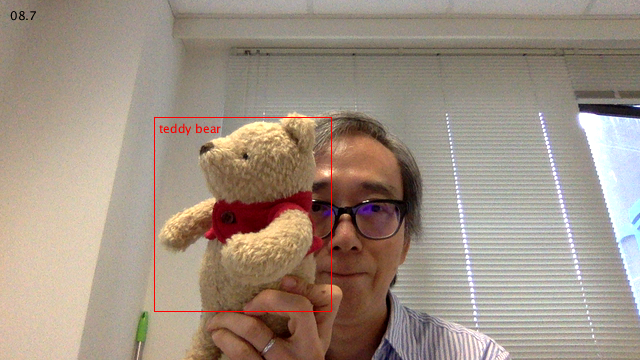# Deep Neural Network (dnn) module with Processing

This is my first demo run of the dnn (deep neural network) module in OpenCV 3.4.2 with Processing, using my CVImage library. The module can input pre-trained models from Caffe, Tensorflow, Darknet, and Torch.  In this example, I used the Tensorflow model Inception v2 SSD COCO from here. I also obtained the label map file from the Tensorflow GitHub. The following 3 files are in the data folder of the Processing sketch.

• frozen_inference_graph.pb
• ssd_inception_v2_coco_2017_11_17.pbtxt
• mscoco_label_map.pbtxt

The source code is in my GitHub repository of this website here.# Tensorflow in Processing with the LabelImage example

I convert the LabelImage example for the Java binding of Tensorflow 1.8.0 to the Processing environment.```import org.tensorflow.Graph; import org.tensorflow.Session; import org.tensorflow.Tensor; import org.tensorflow.TensorFlow;   Graph g1; Output o1; Output o2; Output o3; PFont font; String res;   void setup() { size(640, 480); noLoop(); }   void draw() { background(0); Graph g = new Graph(); String value = "Hello from " + TensorFlow.version(); Tensor t = null; try { t = Tensor.create(value.getBytes("UTF-8")); } catch (Exception e) { println(e.getMessage()); } g.opBuilder("Const", "MyConst") .setAttr("dtype", t.dataType()) .setAttr("value", t) .build(); Session s = new Session(g); Tensor output = null; try { output = s.runner() .fetch("MyConst") .run() .get(0); println(new String(output.bytesValue(), "UTF-8")); } catch (Exception e) { println(e.getMessage()); } }```